﻿ 自同构群的阶为2<sup>t</sup>pq（1≤t≤3）的有限Abel群G

# 自同构群的阶为2tpq（1≤t≤3）的有限Abel群GFinite Abelian Group with Automorphism Group for Order 2tpq（1≤t≤3）

Abstract: In this paper, according to the character of finite Abelian group G and the order of automorphism group of it, the structure of finite Abelian group G with automorphism group for the order 2tpq（1≤t≤3）  is discussed. The following results are obtained: G has 6 types when t = 1; G has 22 types when t = 2; G has 49 types when t = 3.

1. 引言

2. 预备知识

$u=\underset{i,j=1}{\overset{t}{\sum }}{s}_{i}{s}_{j}{m}_{ij}-\underset{i=1}{\overset{t}{\sum }}\frac{{s}_{i}\left({s}_{i}+1\right)}{2},\text{\hspace{0.17em}}\text{\hspace{0.17em}}{m}_{ij}={m}_{\mathrm{max}\left\{i,j\right\}}$

3. 主要结果及证明

(1) 当 $k=0$ 时， $|G|={2}^{{\alpha }_{0}}$。又 ${2}^{{\alpha }_{0}-1}||A\left({S}_{2}\right)|$，而 $|A\left({S}_{2}\right)|||A\left(G\right)|=2pq$，故 ${\alpha }_{0}=1,2$

(I) 当 ${\alpha }_{0}=1$ 时， $|G|=2$，则 $G\cong {C}_{2}$，进而 $|A\left(G\right)|=1$，与 $|A\left(G\right)|=2pq$ 矛盾。

(II) 当 ${\alpha }_{0}=2$ 时， $|G|=4$，则 $G\cong {C}_{{2}^{2}}$${C}_{2}×{C}_{2}$

(i) 若 $G\cong {C}_{{2}^{2}}$，有 $|A\left(G\right)|=2$，与 $|A\left(G\right)|=2pq$ 矛盾。

(ii) 若 $G\cong {C}_{2}×{C}_{2}$，有 $|A\left(G\right)|=6$，与 $|A\left(G\right)|=2pq$ 矛盾。

(2) 当 $k=1$ 时，由于 $2||A\left({S}_{{p}_{i}}\right)|$，得 $A\left({S}_{2}\right)=1$，则有 ${\alpha }_{0}=0,1$。又由 ${p}_{1}^{{\alpha }_{1}-1}\left({p}_{1}-1\right)||A\left({S}_{{p}_{1}}\right)|$，而 $|A\left({S}_{{p}_{1}}\right)|||A\left(G\right)|=2pq$，故 ${\alpha }_{1}=1,2$

(I) 当 ${\alpha }_{1}=1$ 时，则有 ${S}_{{p}_{1}}={C}_{{p}_{1}}$，我们有 $G\cong {C}_{{p}_{1}}$${C}_{2}×{C}_{{p}_{1}}$。由于 $|A\left(G\right)|={p}_{1}-1=2pq$，可得 ${p}_{1}=2pq+1$。当 $2pq+1$ 为素数时，有 ${G}_{1}\cong {C}_{2pq+1}$${G}_{2}\cong {C}_{2}×{C}_{2pq+1}$

(II) 当 ${\alpha }_{1}=2$ 时，则 ${S}_{{p}_{1}}={C}_{{p}_{1}^{2}}$${C}_{{p}_{1}}×{C}_{{p}_{1}}$

(i) 若 ${S}_{{p}_{1}}={C}_{{p}_{1}^{2}}$，有 $G\cong {C}_{{p}_{1}^{2}}$${C}_{2}×{C}_{{p}_{1}^{2}}$。因为 $|A\left(G\right)|={p}_{1}\left({p}_{1}-1\right)=2pq$，令 ${p}_{1}=q$，即 ${p}_{1}=q=2p+1$。当 $2p+1$ 为素数时，有 ${G}_{3}\cong {C}_{{\left(2p+1\right)}^{2}}$${G}_{4}\cong {C}_{2}×{C}_{{\left(2p+1\right)}^{2}}$。同理，令 ${p}_{1}=p$，即 ${p}_{1}=p=2q+1$，当 $2q+1$ 为素数时，有 ${G}_{5}\cong {C}_{{\left(2q+1\right)}^{2}}$${G}_{6}\cong {C}_{2}×{C}_{{\left(2q+1\right)}^{2}}$

(ii) 若 ${S}_{{p}_{1}}={C}_{{p}_{1}}×{C}_{{p}_{1}}$，则有 $G\cong {C}_{{p}_{1}}×{C}_{{p}_{1}}$${C}_{2}×{C}_{{p}_{1}}×{C}_{{p}_{1}}$，此时 $|A\left(G\right)|={p}_{1}{\left({p}_{1}-1\right)}^{2}\left({p}_{1}+1\right)=2pq$，与 ${2}^{3}||A\left(G\right)|=2pq$ 矛盾，故G不存在。

(1) 当 $k=0$ 时， $|G|={2}^{{\alpha }_{0}}$。由于 ${2}^{{\alpha }_{0}-1}||A\left({S}_{2}\right)|$，且 $|A\left({S}_{2}\right)||{2}^{2}pq$，可得 ${\alpha }_{0}=1,2,3$

(I) 当 ${\alpha }_{0}=1,2$ 时，由定理3.1的证明可知，G是不存在的。

(II) 当 ${\alpha }_{0}=3$ 时， $|G|={2}^{3}$，则 $G\cong {C}_{{2}^{3}},{C}_{{2}^{2}}×{C}_{2},{C}_{2}×{C}_{2}×{C}_{2}$

(i) 若 $G\cong {C}_{{2}^{3}}$，有 $|A\left(G\right)|={2}^{2}$

(ii) 若 $G\cong {C}_{{2}^{2}}×{C}_{2}$，有 $|A\left(G\right)|={2}^{3}$

(iii) 若 $G\cong {C}_{2}×{C}_{2}×{C}_{2}$，有 $|A\left(G\right)|={2}^{3}\cdot 3\cdot 7$。均与 $|A\left(G\right)|={2}^{2}pq$ 矛盾。

(2) 当 $k=1$ 时， $|G|={2}^{{\alpha }_{0}}{p}_{1}^{{\alpha }_{1}}$，由 $2||A\left({S}_{{p}_{i}}\right)|$ 可知， $A\left({S}_{2}\right)=1$，则 ${\alpha }_{0}=0,1,2$

(I) 当 ${\alpha }_{0}=0,1$ 时，我们有 ${p}_{1}^{{\alpha }_{1}-1}\left({p}_{1}-1\right)||A\left({S}_{{p}_{1}}\right)|$，并且 $|A\left({S}_{{p}_{1}}\right)|||A\left(G\right)|={2}^{2}pq$，故 ${\alpha }_{1}=1,2$

(i) 当 ${\alpha }_{1}=1$ 时，有 $G\cong {C}_{{p}_{1}},{C}_{2}×{C}_{{p}_{1}}$，此时 $|A\left(G\right)|={p}_{1}-1={2}^{2}pq$，得出 ${p}_{1}={2}^{2}pq+1$。当 ${2}^{2}pq+1$ 为素数时，有 ${G}_{1}\cong {C}_{{2}^{2}pq+1}$${G}_{2}\cong {C}_{2}×{C}_{{2}^{2}pq+1}$

(ii) 当 ${\alpha }_{1}=2$ 时，可知 ${S}_{{p}_{1}}={C}_{{p}_{1}^{2}},{C}_{{p}_{1}}×{C}_{{p}_{1}}$

(a) 若 ，则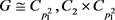。由于 $|A\left(G\right)|={p}_{1}\left({p}_{1}-1\right)={2}^{2}pq$，若令 ${p}_{1}=q$，即 ${p}_{1}=q={2}^{2}p+1$。当 ${2}^{2}p+1$ 为素数时，有 ${G}_{3}\cong {C}_{{\left({2}^{2}p+1\right)}^{2}}$${G}_{4}\cong {C}_{2}×{C}_{{\left({2}^{2}p+1\right)}^{2}}$。同理，令 ${p}_{1}=p$，有 ${G}_{5}\cong {C}_{{\left({2}^{2}q+1\right)}^{2}}$${G}_{6}\cong {C}_{2}×{C}_{{\left({2}^{2}q+1\right)}^{2}}$

(b) 若 ${S}_{{p}_{1}}={C}_{{p}_{1}}×{C}_{{p}_{1}}$，则有 $G\cong {C}_{{p}_{1}}×{C}_{{p}_{1}},{C}_{2}×{C}_{{p}_{1}}×{C}_{{p}_{1}}$，而 $|A\left(G\right)|={p}_{1}{\left({p}_{1}-1\right)}^{2}\left({p}_{1}+1\right)={2}^{2}pq$，与 ${2}^{3}||A\left(G\right)|={2}^{2}pq$ 矛盾，因此G不存在。

(II) 当 ${\alpha }_{0}=2$ 时，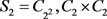(i) 当 ${\alpha }_{1}=1$ 时， ${S}_{{p}_{1}}={C}_{{p}_{1}}$

(a) 若 ${S}_{2}={C}_{{2}^{2}}$，有 $G\cong {C}_{{2}^{2}}×{C}_{{p}_{1}}$，由于 $|A\left(G\right)|=2\left({p}_{1}-1\right)={2}^{2}pq$，可得 ${p}_{1}=2pq+1$。当 $2pq+1$ 为素数时，有 ${G}_{7}\cong {C}_{{2}^{2}}×{C}_{2pq+1}$

(b) 若 ${S}_{2}={C}_{2}×{C}_{2}$，有 $G\cong {C}_{2}×{C}_{2}×{C}_{{p}_{1}}$，因为 $|A\left(G\right)|=2\cdot 3\left({p}_{1}-1\right)={2}^{2}pq$，可令 $p=3$，则有 ${p}_{1}=2q+1$。当 $2q+1$ 为素数时，有 ${G}_{8}\cong {C}_{2}×{C}_{2}×{C}_{2q+1}$

(ii) 当 ${\alpha }_{1}=2$ 时， ${S}_{{p}_{1}}={C}_{{p}_{1}^{2}},{C}_{{p}_{1}}×{C}_{{p}_{1}}$

(a) 若 ${S}_{2}={C}_{{2}^{2}}$，则有 $G\cong {C}_{{2}^{2}}×{C}_{{p}_{1}^{2}},{C}_{{2}^{2}}×{C}_{{p}_{1}}×{C}_{{p}_{1}}$

$G\cong {C}_{{2}^{2}}×{C}_{{p}_{1}^{2}}$，此时 $|A\left(G\right)|=2{p}_{1}\left({p}_{1}-1\right)={2}^{2}pq$。若令 ${p}_{1}=p$，则 ${p}_{1}=p=2q+1$。当 $2q+1$ 为素数时，有 ${G}_{9}\cong {C}_{{2}^{2}}×{C}_{{\left(2q+1\right)}^{2}}$。同理，令 ${p}_{1}=q$ 时，有 ${G}_{10}\cong {C}_{{2}^{2}}×{C}_{{\left(2p+1\right)}^{2}}$

$G\cong {C}_{{2}^{2}}×{C}_{{p}_{1}}×{C}_{{p}_{1}}$，由于 $|A\left(G\right)|=2{p}_{1}{\left({p}_{1}-1\right)}^{2}\left({p}_{1}+1\right)={2}^{2}pq$，得 ${p}_{1}{\left({p}_{1}-1\right)}^{2}\left({p}_{1}+1\right)=2pq$，矛盾，故G不存在。

(b) 若 ${S}_{2}={C}_{2}×{C}_{2}$，此时 $G\cong {C}_{2}×{C}_{2}×{C}_{{p}_{1}^{2}},{C}_{2}×{C}_{2}×{C}_{{p}_{1}}×{C}_{{p}_{1}}$

$G\cong {C}_{2}×{C}_{2}×{C}_{{p}_{1}^{2}}$，由于 $|A\left(G\right)|=2\cdot 3\cdot {p}_{1}\left({p}_{1}-1\right)={2}^{2}pq$，则有 $3\cdot {p}_{1}\left({p}_{1}-1\right)=2pq$，此时得到 ${p}_{1}=p=q=3$，与 $p,q$ 为不同的奇素数矛盾，因此G不存在。

$G\cong {C}_{2}×{C}_{2}×{C}_{{p}_{1}}×{C}_{{p}_{1}}$，由 $|A\left(G\right)|=2\cdot 3\cdot {p}_{1}{\left({p}_{1}-1\right)}^{2}\left({p}_{1}+1\right)={2}^{2}pq$，我们有 $3×{p}_{1}\left({p}_{1}-{1\right)}^{2}\left({p}_{1}+1\right)=2pq$，矛盾，故G不存在。

(3) 当 $k=2$ 时， $|G|={2}^{{\alpha }_{0}}{p}_{1}^{{\alpha }_{1}}{p}_{2}^{{\alpha }_{2}}$，又 $2||A\left({S}_{{p}_{i}}\right)|\left(i=1,2\right)$，所以 ${\alpha }_{0}=0,1$。由 ${p}_{1}^{{\alpha }_{1}-1}\left({p}_{1}-1\right){p}_{2}^{{\alpha }_{2}-1}\left({p}_{2}-1\right)|{2}^{2}pq$，可得 ${\alpha }_{1}=1,2$${\alpha }_{2}=1,2$

(I) ${\alpha }_{1}=1,{\alpha }_{2}=1$$G\cong {C}_{{p}_{1}}×{C}_{{p}_{2}},{C}_{2}×{C}_{{p}_{1}}×{C}_{{p}_{2}}$，此时有 $|A\left(G\right)|=\left({p}_{1}-1\right)\left({p}_{2}-1\right)={2}^{2}pq$。由于 ${p}_{1}-1,{p}_{2}-1$ 是不同的偶数，而 ${2}^{2}pq=2\cdot 2pq=2p\cdot 2q$，则当 $2pq+1$ 为素数时，有 ${G}_{11}\cong {C}_{3}×{C}_{2pq+1}$${G}_{12}\cong {C}_{2}×{C}_{3}×{C}_{2pq+1}$ ；当 $2p+1,2q+1$ 为素数时，有 ${G}_{13}\cong {C}_{2p+1}×{C}_{2q+1}$${G}_{14}\cong {C}_{2}×{C}_{2p+1}×{C}_{2q+1}$

(II) ${\alpha }_{1}=2,{\alpha }_{2}=1$ ：此时只需考虑 $G\cong {C}_{{p}_{1}^{2}}×{C}_{{p}_{2}},{C}_{2}×{C}_{{p}_{1}^{2}}×{C}_{{p}_{2}}$。由于 $|A\left(G\right)|={p}_{1}\left({p}_{1}-1\right)\left({p}_{2}-1\right)={2}^{2}pq$，若令 ${p}_{1}=p$，则有 $\left({p}_{1}-1\right)\left({p}_{2}-1\right)={2}^{2}q$。由于 ${p}_{1}-1,{p}_{2}-1$ 是不同的偶数，而 ${2}^{2}q=2\cdot 2q$，则当 $2q+1$ 为素数时，有 ${G}_{15}\cong {C}_{{3}^{2}}×{C}_{2q+1}$${G}_{16}\cong {C}_{2}×{C}_{{3}^{2}}×{C}_{2q+1}$${G}_{17}\cong {C}_{{\left(2q+1\right)}^{2}}×{C}_{3}$${G}_{18}\cong {C}_{2}×{C}_{{\left(2q+1\right)}^{2}}×{C}_{3}$。同理，令 ${p}_{1}=q$ 时，有 ${G}_{19}\cong {C}_{{3}^{2}}×{C}_{2p+1}$${G}_{20}\cong {C}_{2}×{C}_{{3}^{2}}×{C}_{2p+1}$${G}_{21}\cong {C}_{{\left(2p+1\right)}^{2}}×{C}_{3}$${G}_{22}\cong {C}_{2}×{C}_{{\left(2p+1\right)}^{2}}×{C}_{3}$

(III) ${\alpha }_{1}=2,{\alpha }_{2}=2$ ：只需考虑 $G\cong {C}_{{p}_{1}^{2}}×{C}_{{p}_{2}^{2}},{C}_{2}×{C}_{{p}_{1}^{2}}×{C}_{{p}_{2}^{2}}$，由 $|A\left(G\right)|={p}_{1}\left({p}_{1}-1\right){p}_{2}\left({p}_{2}-1\right)={2}^{2}pq$，可得 ${p}_{1}={p}_{2}=p=q=3$，与 $p,q$ 为互异的奇素数不符，故G不存在。

(1) 当 $k=0$ 时， $|G|={2}^{{\alpha }_{0}}$。又因为 ${2}^{{\alpha }_{0}-1}||A\left({S}_{2}\right)|$，而 $|A\left({S}_{2}\right)||{2}^{3}pq$，所以 ${\alpha }_{0}=1,2,3,4$

(I) 当 ${\alpha }_{0}=1,2,3$ 时，由定理3.2的证明知，G不存在。

(II) 当 ${\alpha }_{0}=4$ 时， $|G|={2}^{4}$，则 $G\cong {C}_{{2}^{4}},{C}_{{2}^{3}}×{C}_{2},{C}_{{2}^{2}}×{C}_{2}×{C}_{2},{C}_{{2}^{2}}×{C}_{{2}^{2}},{C}_{2}×{C}_{2}×{C}_{2}×{C}_{2}$

(i) 若 $G\cong {C}_{{2}^{4}}$，则有 $|A\left(G\right)|={2}^{3}$${2}^{3}pq$ 不符。

(ii) 若 $G\cong {C}_{{2}^{3}}×{C}_{2},{C}_{{2}^{2}}×{C}_{2}×{C}_{2},{C}_{{2}^{2}}×{C}_{{2}^{2}},{C}_{2}×{C}_{2}×{C}_{2}×{C}_{2}$，由计算可知均有  矛盾，故G不存在。

(2) 当 $k=1$ 时， $|G|={2}^{{\alpha }_{0}}{p}_{1}^{{\alpha }_{1}}$，因为 $2||A\left({S}_{{p}_{i}}\right)|$，可知 $A\left({S}_{2}\right)=1$，则 ${\alpha }_{0}=0,1,2,3$

(I) 当 ${\alpha }_{0}=0,1$ 时，由于 ${p}_{1}^{{\alpha }_{1}-1}\left({p}_{1}-1\right)||A\left({S}_{{p}_{1}}\right)|$，而又有 $|A\left({S}_{{p}_{1}}\right)|||A\left(G\right)|={2}^{3}pq$，故 ${\alpha }_{1}=1,2$

(i) 当 ${\alpha }_{1}=1$ 时， $G\cong {C}_{{p}_{1}},{C}_{2}×{C}_{{p}_{1}}$，此时 $|A\left(G\right)|={p}_{1}-1={2}^{3}pq$，得到 ${p}_{1}={2}^{3}pq+1$。当 ${2}^{3}pq+1$ 为素数时，有 ${G}_{1}\cong {C}_{{2}^{3}pq+1}$${G}_{2}\cong {C}_{2}×{C}_{{2}^{3}pq+1}$

(ii) 当 ${\alpha }_{1}=2$ 时，可知 ${S}_{{p}_{1}}={C}_{{p}_{1}^{2}},{C}_{{p}_{1}}×{C}_{{p}_{1}}$

(a) 若 ${S}_{{p}_{1}}={C}_{{p}_{1}^{2}}$，有 $G\cong {C}_{{p}_{1}^{2}},{C}_{2}×{C}_{{p}_{1}^{2}}$，此时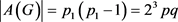。若令 ${p}_{1}=q$，即 ${p}_{1}=q={2}^{3}p+1$。当 ${2}^{3}p+1$ 为素数时，有 ${G}_{3}\cong {C}_{{\left({2}^{3}p+1\right)}^{2}}$${G}_{4}\cong {C}_{2}×{C}_{{\left({2}^{3}p+1\right)}^{2}}$。同理，令 ${p}_{1}=p$ 时，有 ${G}_{5}\cong {C}_{{\left({2}^{3}q+1\right)}^{2}}$${G}_{6}\cong {C}_{2}×{C}_{{\left({2}^{3}q+1\right)}^{2}}$

(b) 若 ${S}_{{p}_{1}}={C}_{{p}_{1}}×{C}_{{p}_{1}}$，则有 $G\cong {C}_{{p}_{1}}×{C}_{{p}_{1}},{C}_{2}×{C}_{{p}_{1}}×{C}_{{p}_{1}}$。由 $|A\left(G\right)|={p}_{1}{\left({p}_{1}-1\right)}^{2}\left({p}_{1}+1\right)={2}^{3}pq$，若 ${p}_{1}-1=2\lambda$$\lambda$ 为偶数时，产生矛盾。 $\lambda$ 为奇数时，代入等式左边为偶数，右边为奇数，不符，因此G不存在。

(II) 当 ${\alpha }_{0}=2$ 时， ${S}_{2}={C}_{{2}^{2}},{C}_{2}×{C}_{2}$

(i) 当 ${\alpha }_{1}=1$ 时， ${S}_{{p}_{1}}={C}_{{p}_{1}}$

(a) 若 ${S}_{2}={C}_{{2}^{2}}$，有 $G\cong {C}_{{2}^{2}}×{C}_{{p}_{1}}$，由 $|A\left(G\right)|=2\left({p}_{1}-1\right)={2}^{3}pq$，得出 ${p}_{1}={2}^{2}pq+1$。当 ${2}^{2}pq+1$ 为素数时，有 ${G}_{7}\cong {C}_{{2}^{2}}×{C}_{{2}^{2}pq+1}$

(b) 若 ${S}_{2}={C}_{2}×{C}_{2}$，则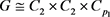，此时 $|A\left(G\right)|=2\cdot 3\left({p}_{1}-1\right)={2}^{3}pq$。若 $p=3$，可知 ${p}_{1}={2}^{2}q+1$。当 ${2}^{2}q+1$ 为素数时，有 ${G}_{8}\cong {C}_{2}×{C}_{2}×{C}_{{2}^{2}q+1}$

(ii) 当 ${\alpha }_{1}=2$ 时， ${S}_{{p}_{1}}={C}_{{p}_{1}^{2}},{C}_{{p}_{1}}×{C}_{{p}_{1}}$

(a) 若 ${S}_{2}={C}_{{2}^{2}}$，可知 $G\cong {C}_{{2}^{2}}×{C}_{{p}_{1}^{2}},{C}_{{2}^{2}}×{C}_{{p}_{1}}×{C}_{{p}_{1}}$

$G\cong {C}_{{2}^{2}}×{C}_{{p}_{1}^{2}}$，由 $|A\left(G\right)|=2{p}_{1}\left({p}_{1}-1\right)={2}^{3}pq$，得 ${p}_{1}\left({p}_{1}-1\right)={2}^{2}pq$。若令 ${p}_{1}=p$，即 ${p}_{1}=p={2}^{2}q+1$。当 ${2}^{2}q+1$ 为素数时，有 ${G}_{9}\cong {C}_{{2}^{2}}×{C}_{{\left({2}^{2}q+1\right)}^{2}}$。同理，令 ${p}_{1}=q$ 时，有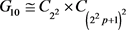$G\cong {C}_{{2}^{2}}×{C}_{{p}_{1}}×{C}_{{p}_{1}}$，由 $|A\left(G\right)|=2{p}_{1}{\left({p}_{1}-1\right)}^{2}\left({p}_{1}+1\right)={2}^{3}pq$，得 ${p}_{1}{\left({p}_{1}-1\right)}^{2}\left({p}_{1}+1\right)={2}^{2}pq$，产生矛盾，故G不存在。

(b) 若 ${S}_{2}={C}_{2}×{C}_{2}$，有 $G\cong {C}_{2}×{C}_{2}×{C}_{{p}_{1}^{2}}$${C}_{2}×{C}_{2}×{C}_{{p}_{1}}×{C}_{{p}_{1}}$

$G\cong {C}_{2}×{C}_{2}×{C}_{{p}_{1}^{2}}$，我们有 $|A\left(G\right)|=2\cdot 3\cdot {p}_{1}\left({p}_{1}-1\right)={2}^{3}pq$，从而 $3\cdot {p}_{1}\left({p}_{1}-1\right)={2}^{2}pq$。若令 $p=3$，则 ，于是有 ${G}_{11}\cong {C}_{2}×{C}_{2}×{C}_{{5}^{2}}$

$G\cong {C}_{2}×{C}_{2}×{C}_{{p}_{1}}×{C}_{{p}_{1}}$，则有 $|A\left(G\right)|=2\cdot 3\cdot {p}_{1}{\left({p}_{1}-1\right)}^{2}\left({p}_{1}+1\right)={2}^{3}pq$，于是 $3\cdot {p}_{1}\left({p}_{1}-{1\right)}^{2}\left({p}_{1}+1\right)={2}^{2}pq$，产生矛盾，因此G不存在。

(III) 当 ${\alpha }_{0}=3$ 时， ${S}_{2}={C}_{{2}^{3}},{C}_{{2}^{2}}×{C}_{2},{C}_{2}×{C}_{2}×{C}_{2}$。当 ${S}_{2}={C}_{{2}^{2}}×{C}_{2},{C}_{2}×{C}_{2}×{C}_{2}$ 时，由前面的证明易知G不存在，所以只需考虑 ${S}_{2}={C}_{{2}^{3}}$

(i) 当 ${\alpha }_{1}=1$ 时， ${S}_{{p}_{1}}={C}_{{p}_{1}}$，有 $G\cong {C}_{{2}^{3}}×{C}_{{p}_{1}}$。由于 $|A\left(G\right)|={2}^{2}\left({p}_{1}-1\right)={2}^{3}pq$，可得 ${p}_{1}-1=2pq$，从而 ${p}_{1}=2pq+1$。当 $2pq+1$ 为素数时，有 ${G}_{12}\cong {C}_{{2}^{3}}×{C}_{2pq+1}$

(ii) 当 ${\alpha }_{1}=2$ 时， ${S}_{{p}_{1}}={C}_{{p}_{1}^{2}},{C}_{{p}_{1}}×{C}_{{p}_{1}}$，同样也只考虑 ${S}_{{p}_{1}}={C}_{{p}_{1}^{2}}$，于是 $G\cong {C}_{{2}^{3}}×{C}_{{p}_{1}^{2}}$，此时 $|A\left(G\right)|={2}^{2}{p}_{1}\left({p}_{1}-1\right)={2}^{3}pq$，可得 ${p}_{1}\left({p}_{1}-1\right)=2pq$。若令 ${p}_{1}=p$，即 ${p}_{1}=p=2q+1$，当 $2q+1$ 为素数时，有 ${G}_{13}\cong {C}_{{2}^{3}}×{C}_{{\left(2q+1\right)}^{2}}$。同理，令 ${p}_{1}=q$ 时，有 ${G}_{14}\cong {C}_{{2}^{3}}×{C}_{{\left(2p+1\right)}^{2}}$

(3) 当 $k=2$ 时， $|G|={2}^{{\alpha }_{0}}{p}_{1}^{{\alpha }_{1}}{p}_{2}^{{\alpha }_{2}}$，又因为 $2||A\left({S}_{{p}_{i}}\right)|\left(i=1,2\right)$，所以 ${\alpha }_{0}=0,1,2$。由 ${p}_{1}^{{\alpha }_{1}-1}\left({p}_{1}-1\right){p}_{2}^{{\alpha }_{2}-1}\left({p}_{2}-1\right)|{2}^{3}pq$，得出 ${\alpha }_{1}=1,2$${\alpha }_{2}=1,2$

(I) 当 ${\alpha }_{0}=0,1$ 时，

(i) ${\alpha }_{1}=1,{\alpha }_{2}=1$ ：此时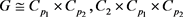，于是 $|A\left(G\right)|=\left({p}_{1}-1\right)\left({p}_{2}-1\right)={2}^{3}pq$。由于 ${p}_{1}-1,{p}_{2}-1$ 是不同的偶数，而 ${2}^{3}pq=2\cdot {2}^{2}pq={2}^{2}\cdot 2pq=2p\cdot {2}^{2}q={2}^{2}p\cdot 2q$。所以当 ${2}^{2}pq+1$ 为素数时，有 ${G}_{15}\cong {C}_{3}×{C}_{{2}^{2}pq+1}$${G}_{16}\cong {C}_{2}×{C}_{3}×{C}_{{2}^{2}pq+1}$ ；当 $2pq+1$ 为素数时，有 ${G}_{17}\cong {C}_{5}×{C}_{2pq+1}$${G}_{18}\cong {C}_{2}×{C}_{5}×{C}_{2pq+1}$ ；当 $2p+1,{2}^{2}q+1$ 为素数时，有 ${G}_{19}\cong {C}_{2p+1}×{C}_{{2}^{2}q+1}$${G}_{20}\cong {C}_{2}×{C}_{2p+1}×{C}_{{2}^{2}q+1}$ ；当 ${2}^{2}p+1,2q+1$ 为素数时，有 ${G}_{21}\cong {C}_{{2}^{2}p+1}×{C}_{2q+1}$${G}_{22}\cong {C}_{2}×{C}_{{2}^{2}p+1}×{C}_{2q+1}$

(ii) ${\alpha }_{1}=2,{\alpha }_{2}=1$ ：只需考虑 $G\cong {C}_{{p}_{1}^{2}}×{C}_{{p}_{2}},{C}_{2}×{C}_{{p}_{1}^{2}}×{C}_{{p}_{2}}$，于是有 $|A\left(G\right)|={p}_{1}\left({p}_{1}-1\right)\left({p}_{2}-1\right)={2}^{3}pq$。由于 ${p}_{1}-1,{p}_{2}-1$ 是不同的偶数，若 ${p}_{1}=p$，则 $\left(p-1\right)\left({p}_{2}-1\right)={2}^{3}q$，而 ${2}^{3}q=2\cdot {2}^{2}q={2}^{2}\cdot 2q$。所以当 ${2}^{2}q+1$ 为素数时，有${G}_{24}\cong {C}_{2}×{C}_{{3}^{2}}×{C}_{{2}^{2}q+1}$${G}_{25}\cong {C}_{{\left({2}^{2}q+1\right)}^{2}}×{C}_{3}$${G}_{26}\cong {C}_{2}×{C}_{{\left({2}^{2}q+1\right)}^{2}}×{C}_{3}$ ；当 $2q+1$ 为素数时，有 ${G}_{27}\cong {C}_{{5}^{2}}×{C}_{2q+1}$${G}_{28}\cong {C}_{2}×{C}_{{5}^{2}}×{C}_{2q+1}$${G}_{29}\cong {C}_{{\left(2q+1\right)}^{2}}×{C}_{5}$${G}_{30}\cong {C}_{2}×{C}_{{\left(2q+1\right)}^{2}}×{C}_{5}$。同理，若令 ${p}_{1}=q$，则当  为素数时，有 ${G}_{31}\cong {C}_{{3}^{2}}×{C}_{{2}^{2}p+1}$${G}_{32}\cong {C}_{2}×{C}_{{3}^{2}}×{C}_{{2}^{2}p+1}$${G}_{33}\cong {C}_{{\left({2}^{2}p+1\right)}^{2}}×{C}_{3}$${G}_{34}\cong {C}_{2}×{C}_{{\left({2}^{2}p+1\right)}^{2}}×{C}_{3}$ ；当 $2p+1$ 为素数时，有 ${G}_{35}\cong {C}_{{5}^{2}}×{C}_{2p+1}$${G}_{36}\cong {C}_{2}×{C}_{{5}^{2}}×{C}_{2p+1}$${G}_{37}\cong {C}_{{\left(2p+1\right)}^{2}}×{C}_{5}$${G}_{38}\cong {C}_{2}×{C}_{{\left(2p+1\right)}^{2}}×{C}_{5}$

(iii) ${\alpha }_{1}=2,{\alpha }_{2}=2$ ：我们只需考虑 $G\cong {C}_{{p}_{1}^{2}}×{C}_{{p}_{2}^{2}},{C}_{2}×{C}_{{p}_{1}^{2}}×{C}_{{p}_{2}^{2}}$，由 $|A\left(G\right)|={p}_{1}\left({p}_{1}-1\right){p}_{2}\left({p}_{2}-1\right)={2}^{3}pq$，可得 ${p}_{1}=p=3$${p}_{2}=q=5$，则有 ${G}_{39}\cong {C}_{{3}^{2}}×{C}_{{5}^{2}}$${G}_{40}\cong {C}_{2}×{C}_{{3}^{2}}×{C}_{{5}^{2}}$

(II) 当 ${\alpha }_{0}=2$ 时， ${S}_{2}={C}_{{2}^{2}},{C}_{2}×{C}_{2}$

(i) 当 ${\alpha }_{1}=1,{\alpha }_{2}=1$ 时，

(a) 若 ${S}_{2}={C}_{{2}^{2}}$，有 $G\cong {C}_{{2}^{2}}×{C}_{{p}_{1}}×{C}_{{p}_{2}}$，从而 $|A\left(G\right)|=2\left({p}_{1}-1\right)\left({p}_{2}-1\right)={2}^{3}pq$，进而 $\left({p}_{1}-1\right)\left({p}_{2}-1\right)={2}^{2}pq$。由于 ${p}_{1}-1,{p}_{2}-1$ 是不同的偶数，而 ${2}^{2}pq=2\cdot 2pq=2p\cdot 2q$。所以当 $2pq+1$ 为素数时，有 ${G}_{41}\cong {C}_{{2}^{2}}×{C}_{3}×{C}_{2pq+1}$ ；当 $2p+1,2q+1$ 为素数时，有 ${G}_{42}\cong {C}_{{2}^{2}}×{C}_{2p+1}×{C}_{2q+1}$

(b) 若 ${S}_{2}={C}_{2}×{C}_{2}$，有 $G\cong {C}_{2}×{C}_{2}×{C}_{{p}_{1}}×{C}_{{p}_{2}}$，从而 $|A\left(G\right)|=2\cdot 3\left({p}_{1}-1\right)\left({p}_{2}-1\right)={2}^{3}pq$，进而 $3\left({p}_{1}-1\right)\left({p}_{2}-1\right)={2}^{2}pq$。若令 $p=3$，则有 $\left({p}_{1}-1\right)\left({p}_{2}-1\right)={2}^{2}q$，而 ${2}^{2}q=2\cdot 2q$。所以当 $2q+1$ 为素数时，有 ${G}_{43}\cong {C}_{2}×{C}_{2}×{C}_{3}×{C}_{2q+1}$

(ii) 当 ${\alpha }_{1}=2,{\alpha }_{2}=1$ 时，

(a) 若 ${S}_{2}={C}_{{2}^{2}}$，我们有 $G\cong {C}_{{2}^{2}}×{C}_{{p}_{1}^{2}}×{C}_{{p}_{2}}$，从而 $|A\left(G\right)|=2{p}_{1}\left({p}_{1}-1\right)\left({p}_{2}-1\right)={2}^{3}pq$，进而 ${p}_{1}\left({p}_{1}-1\right)\left({p}_{2}-1\right)={2}^{2}pq$。若 ${p}_{1}=p$，则 $\left(p-1\right)\left({p}_{2}-1\right)={2}^{2}q$，而 ${2}^{2}q=2\cdot 2q$。当 $2q+1$ 为素数时，有 ${G}_{44}\cong {C}_{{2}^{2}}×{C}_{{3}^{2}}×{C}_{2q+1}$${G}_{45}\cong {C}_{{2}^{2}}×{C}_{{\left(2q+1\right)}^{2}}×{C}_{3}$ ；同理，若令 ${p}_{1}=q$，则有 ${G}_{46}\cong {C}_{{2}^{2}}×{C}_{{3}^{2}}×{C}_{2p+1}$${G}_{47}\cong {C}_{{2}^{2}}×{C}_{{\left(2p+1\right)}^{2}}×{C}_{3}$

(b) 若 ${S}_{2}={C}_{2}×{C}_{2}$，有 $G\cong {C}_{2}×{C}_{2}×{C}_{{p}_{1}^{2}}×{C}_{{p}_{2}}$。由于 $|A\left(G\right)|=2\cdot 3{p}_{1}\left({p}_{1}-1\right)\left({p}_{2}-1\right)={2}^{3}pq$，从而 $3{p}_{1}\left({p}_{1}-1\right)\left({p}_{2}-1\right)={2}^{2}pq$。此时 ${p}_{1}={p}_{2}=p=q=3$，与 $p,q$ 为互异的奇素数不符，故G不存在。

(iii) 当 ${\alpha }_{1}=2,{\alpha }_{2}=2$ 时，

(a) 则 $G\cong {C}_{{2}^{2}}×{C}_{{p}_{1}^{2}}×{C}_{{p}_{2}^{2}}$，于是 $|A\left(G\right)|=2{p}_{1}\left({p}_{1}-1\right){p}_{2}\left({p}_{2}-1\right)={2}^{3}pq$，可得 ${p}_{1}\left({p}_{1}-1\right){p}_{2}\left({p}_{2}-1\right)={2}^{2}pq$。此时 ${p}_{1}={p}_{2}=p=q=3$，与 $p,q$ 为不同的奇素数矛盾，所以G不存在。

(b) 有 $G\cong {C}_{2}×{C}_{2}×{C}_{{p}_{1}^{2}}×{C}_{{p}_{2}^{2}}$，此时 $|A\left(G\right)|=2\cdot 3{p}_{1}\left({p}_{1}-1\right){p}_{2}\left({p}_{2}-1\right)={2}^{3}pq$，从而可得 $3{p}_{1}\left({p}_{1}-1\right){p}_{2}\left({p}_{2}-1\right)={2}^{2}pq$，矛盾，所以G不存在。

(4) 当 $k=3$ 时，我们有 ${\alpha }_{0}=0,1$

(I)  ：此时 $G\cong {C}_{{p}_{1}}×{C}_{{p}_{2}}×{C}_{{p}_{3}},{C}_{2}×{C}_{{p}_{1}}×{C}_{{p}_{2}}×{C}_{{p}_{3}}$，从而 $|A\left(G\right)|=\left({p}_{1}-1\right)\left({p}_{2}-1\right)\left({p}_{3}-1\right)={2}^{3}pq$，而 ${2}^{3}pq=2\cdot 2p\cdot 2q$。所以当 $2p+1,2q+1$ 为素数时，有 ${G}_{48}\cong {C}_{3}×{C}_{2p+1}×{C}_{2q+1}$${G}_{49}\cong {C}_{2}×{C}_{3}×{C}_{2p+1}×{C}_{2q+1}$

(II) ${\alpha }_{1}=2,{\alpha }_{2}=1,{\alpha }_{3}=1$ ：此时 $G\cong {C}_{{p}_{1}^{2}}×{C}_{{p}_{2}}×{C}_{{p}_{3}},{C}_{2}×{C}_{{p}_{1}^{2}}×{C}_{{p}_{2}}×{C}_{{p}_{3}}$，从而 $|A\left(G\right)|={p}_{1}\left({p}_{1}-1\right)\left({p}_{2}-1\right)\left({p}_{3}-1\right)={2}^{3}pq$。令 ${p}_{1}=p$，而 ${2}^{3}q=2\cdot 2\cdot 2q$，此时 ${p}_{1}={p}_{2}=p=3$${p}_{3}=2q+1$，产生矛盾，因此G不存在。同理， ${p}_{1}=q$ 时，G也是不存在的。

(III) ${\alpha }_{1}=2,{\alpha }_{2}=2,{\alpha }_{3}=1$ ：有 $G\cong {C}_{{p}_{1}^{2}}×{C}_{{p}_{2}^{2}}×{C}_{{p}_{3}},{C}_{2}×{C}_{{p}_{1}^{2}}×{C}_{{p}_{2}^{2}}×{C}_{{p}_{3}}$。由于 $|A\left(G\right)|={p}_{1}\left({p}_{1}-1\right){p}_{2}\left({p}_{2}-1\right)\left({p}_{3}-1\right)={2}^{3}pq$，此时得到 ${p}_{1}={p}_{2}={p}_{3}=p=q=3$，与 $p,q$ 为互异的奇素数不符，从而G不存在。

(IV) ${\alpha }_{1}=2,{\alpha }_{2}=2,{\alpha }_{3}=2$ ：有 $G\cong {C}_{{p}_{1}^{2}}×{C}_{{p}_{2}^{2}}×{C}_{{p}_{3}^{2}},{C}_{2}×{C}_{{p}_{1}^{2}}×{C}_{{p}_{2}^{2}}×{C}_{{p}_{3}^{2}}$，此时 $|A\left(G\right)|={p}_{1}\left({p}_{1}-1\right){p}_{2}\left({p}_{2}-1\right){p}_{3}\left({p}_{3}-1\right)={2}^{3}pq$，产生矛盾，故G不存在。

4. 结束语

 余红宴, 黄本文.自同构群的阶为2tp2q（t=1,2,3）的有限Abel群G [J]. 数学杂志, 2010, 30(5): 883-890.

 余红宴. 自同构群的阶为2tp2(p为奇素数)的有限Abel群G [J]. 信阳师范学院学报（自然科学版）, 2011, 24(3): 287-291.

 黄本文.|A(G)|=2tpqr（1≤t≤3）的有限Abel群G的构造[J]. 武汉大学学报(自然科学版), 1993(2): 9-13.

 张远达. 有限群构造(上、下册) [M]. 北京: 科学出版社, 1982.

 俞曙霞. 有限交换p-群的自同构群的阶的几点注记[J]. 数学杂志, 1983(2): 189-194.

Top